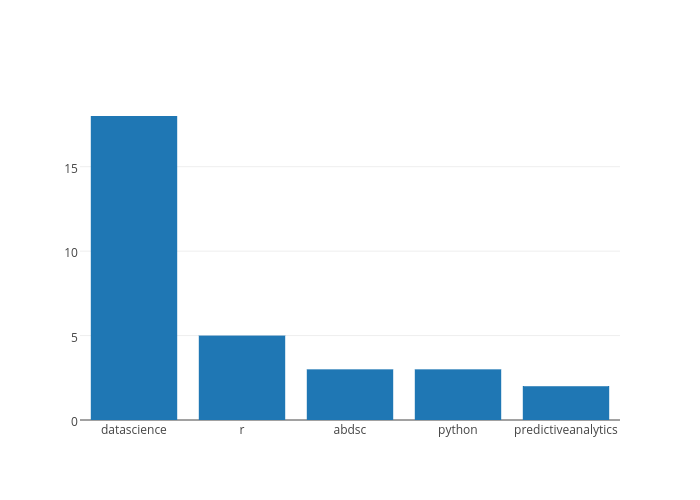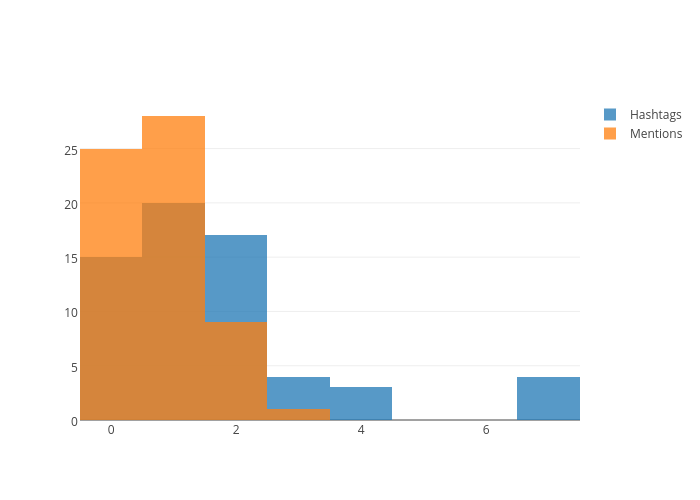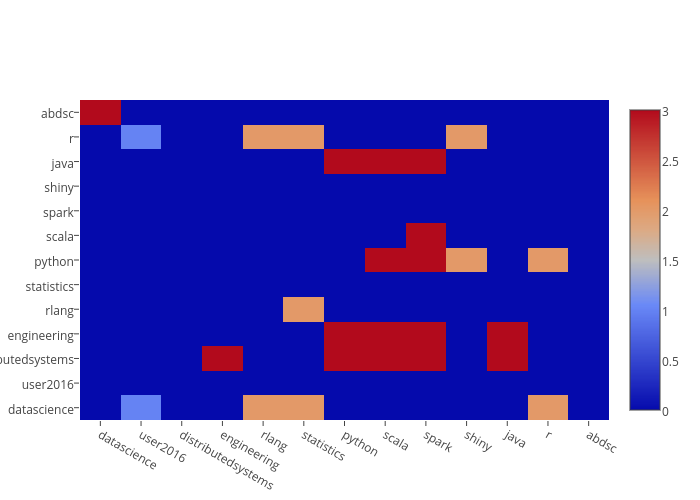# Example Project¶

We're going to import Twitter data into Neo4j and then do various analysis on top of this dataset including plotting, graph algorithms, and graph visualizations.

• Import
• Plotting / Charting
• Graph Algorithms
• Graph Visualization

# Import¶

The script for importing data from Twitter's API is in scripts/twitter.py. This was used to upload tweets that contained either the words "ddtx16" or "datadaytexas" to Neo4j.

## Packages¶

In :
import os
import sys
import time
import requests
from py2neo import Graph, Node, Relationship


## Uniqueness Constraints¶

Neo4j supports uniqueness constraints on given label, property pairs.

In [ ]:
graph = Graph()

graph.run("CREATE CONSTRAINT ON (u:User) ASSERT u.username IS UNIQUE")
graph.run("CREATE CONSTRAINT ON (t:Tweet) ASSERT t.id IS UNIQUE")
graph.run("CREATE CONSTRAINT ON (h:Hashtag) ASSERT h.name IS UNIQUE")


In [ ]:
TWITTER_BEARER = os.environ["TWITTER_BEARER"]

count=100,
result_type="recent",
lang="en",
q=sys.argv
)



## Find Tweets¶

In :
def find_tweets(since_id):

tweets = r.json()["statuses"]

return tweets


In :
def upload_tweets(tweets):
for t in tweets:
u = t["user"]
e = t["entities"]

tweet = Node("Tweet", id=t["id"])
graph.merge(tweet)
tweet["text"] = t["text"]
tweet.push()

graph.merge(user)

graph.merge(Relationship(user, "POSTS", tweet))

for h in e.get("hashtags", []):
hashtag = Node("Hashtag", name=h["text"].lower())
graph.merge(hashtag)
graph.merge(Relationship(hashtag, "TAGS", tweet))

for m in e.get('user_mentions', []):
graph.merge(mention)
graph.merge(Relationship(tweet, "MENTIONS", mention))

ret = t.get("retweeted_status", {}).get("id")

if ret:
retweet = Node("Tweet", id=ret)
graph.merge(retweet)
graph.merge(Relationship(tweet, "RETWEETS", retweet))


## Run It!¶

In [ ]:
since_id = -1

while True:
try:
tweets = find_tweets(since_id=since_id)

if not tweets:
print("No tweets found.")
time.sleep(60)
continue

since_id = tweets.get("id")

time.sleep(60)

except Exception as e:
print(e)
time.sleep(60)
continue


## Visualize the Model¶

In :
from scripts.vis import draw
options = {"User": "username", "Hashtag": "name"}

draw(graph, options, physics=True, limit=30)

Out:

# Plotting¶

Let's gather some basic insights by creating plotly charts.

## Packages¶

In :
%load_ext cypher
import plotly.plotly as py
from plotly.graph_objs import *


## What are the top hashtags?¶

Find hashtags ordered by the number of tweets they've tagged.

In :
result = %cypher MATCH (hashtag:Hashtag)-[:TAGS]->(tweet:Tweet)         \
WHERE hashtag.name <> 'rstats'                         \
RETURN hashtag.name AS hashtag, count(tweet) AS tweets \
ORDER BY tweets DESC LIMIT 5

df = result.get_dataframe()

5 rows affected.

Out:
hashtag tweets
0 datascience 18
1 r 5
2 abdsc 3
3 python 3
4 predictiveanalytics 2
In :
data = Data([Bar(x=df["hashtag"], y=df["tweets"])])

py.image.ishow({'data': data})## What's the hashtag and mention count distribution?¶

In :
result = %cypher MATCH (tweet:Tweet)                                   \
RETURN tweet.id,                                      \
size((:Hashtag)-[:TAGS]->(tweet)) AS hashtags, \
size((tweet)-[:MENTIONS]->(:User)) AS mentions

df = result.get_dataframe()
del df["tweet.id"]

63 rows affected.

Out:
hashtags mentions
0 0 0
1 1 2
2 0 0
3 1 0
4 2 1
In :
hashtags = Histogram(x=df["hashtags"], opacity=0.75, name="Hashtags")
mentions = Histogram(x=df["mentions"], opacity=0.75, name="Mentions")
data = Data([hashtags, mentions])
layout = Layout(barmode="overlay")
fig = Figure(data=data, layout=layout)

py.image.ishow(fig)## Heatmap of hashtag co-occurrence¶

In :
result = %cypher MATCH (h:Hashtag)                           \
WHERE h.name <> "rstats"                    \
WITH h, size((h)-[:TAGS]->(:Tweet)) AS tags \
ORDER BY tags DESC                          \
LIMIT 15                                    \
\
WITH collect(h) AS top_hash                 \
UNWIND top_hash AS h1                       \
UNWIND top_hash AS h2                       \
\
MATCH (h1)-[:TAGS]->(:Tweet)<-[:TAGS]-(h2)  \
WHERE h1.name < h2.name                     \
RETURN h1.name, h2.name, count(*) AS weight

df = result.get_dataframe()

27 rows affected.

Out:
h1.name h2.name weight
0 distributedsystems java 3
1 abdsc datascience 3
2 java python 3
3 datascience r 2
4 java spark 3
In :
names = list(set(list(df["h1.name"]) + list(df["h2.name"])))
heat = [[0 for i in range(len(names))] for j in range(len(names))]

for idx, row in df.iterrows():
i = names.index(row["h1.name"])
j = names.index(row["h2.name"])
heat[i][j] = row["weight"]

In :
import plotly.graph_objs as go

data = [go.Heatmap(z = heat, x = names, y = names)]
py.image.ishow({'data': data})# Graph Algorithms¶

The typical workflow consists of retrieving a subgraph from Neo4j via Cypher and analyzing this graph in igraph.

## A subgraph of users retweeting other users¶

In :
from igraph import Graph as IGraph

query = """
MATCH (user1:User)-[:POSTS]->(retweet:Tweet)-[:RETWEETS]->(tweet:Tweet),
(user2:User)-[:POSTS]->(tweet)
"""

data = graph.run(query)

ig = IGraph.TupleList(data, weights=True)
ig

Out:
<igraph.Graph at 0x10e4437c8>

### Which users have the highest betweenness?¶

$betweenness(v) = \sum_{s, t \in V} \frac{\sigma_{st}(v)}{\sigma_{st}}$

The betweenness centrality of a node $v$ is the number of shortest paths that pass through $v$, $\sigma_{st}(v)$, divided by the total number of shortest paths, $\sigma_{st}$.

In :
between = [(node["name"], node.betweenness()) for node in ig.vs]
top = sorted(between, key=lambda x: x, reverse=True)
top[:5]

Out:
[('revodavid', 10.0),
('Rbloggers', 6.0),
('raymondpeck3', 1.0),
('kuanhoong', 0.0),
('ozjimbob', 0.0)]

### Which users have the highest closeness?¶

$closeness(v) = \frac{1}{\sum_{x} d(v, x)}$

The closeness centrality is the reciprocal of a node's farness, or sum of its shortest path distances from all other nodes in the graph.

In :
close = [(node["name"], node.closeness()) for node in ig.vs]
top = sorted(close, key=lambda x: x, reverse=True)
top[:5]

Out:
[('revodavid', 0.09090909090909091),
('kuanhoong', 0.08875739644970414),
('josefslerka', 0.08875739644970414),
('permutans', 0.08875739644970414),
('HoloMarkeD', 0.08875739644970414)]

### Community detection¶

In :
clusters = IGraph.community_walktrap(ig, weights="weight")
clusters = clusters.as_clustering()
len(clusters)

Out:
4
In :
nodes = [{"id": node.index, "label": node["name"]} for node in ig.vs]

for node in nodes:
node["group"] = clusters.membership[node["id"]]

nodes[:5]

Out:
[{'group': 0, 'id': 0, 'label': 'kuanhoong'},
{'group': 0, 'id': 1, 'label': 'revodavid'},
{'group': 1, 'id': 2, 'label': 'ozjimbob'},
{'group': 1, 'id': 3, 'label': 'Rbloggers'},
{'group': 0, 'id': 4, 'label': 'josefslerka'}]
In :
edges = [{"from": x, "to": x} for x in ig.get_edgelist()]
edges[:5]

Out:
[{'from': 0, 'to': 1},
{'from': 2, 'to': 3},
{'from': 1, 'to': 4},
{'from': 5, 'to': 6},
{'from': 6, 'to': 7}]
In :
from scripts.vis import vis_network
vis_network(nodes, edges, physics=True)

Out:

# Graph Visualization¶

## jgraph¶

Let's visualize (in 3D!) the structure of users retweeting and replying to other users.

In :
import jgraph

query = """